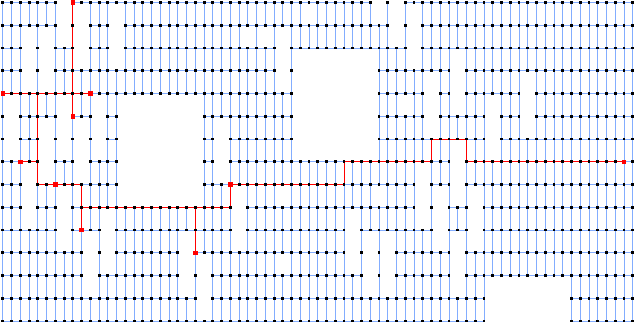# Testset GAP

These instances are grid graphs with rectangular holes. They come from a VLSI application (see JMRW94 ).

They were introduced in KM98 . More information can be found among others in UdAR99 and PD00 .

Here is an image of alue7080 PS DjVu with solution made by E. Uchoa .

This is a picture of diw0495 with solution.Name   |V|    |E|    |T|    DC    Opt
gap1307342 552 17 Ls 549
gap1413541 906 10 Ls 457
gap1500220 374 17 Ls 254
gap1810429 702 17 Ls 482
gap1904735 1256 21 Ps 763
gap20072039 3548 17 NPs 1104
gap21191724 2975 29 Ls 1244
gap27401196 2084 14 Ps 745
gap2800386 653 12 Ls 386
gap2975179 293 10 Ls 245
gap3036346 583 13 Ps 457
gap3100921 1558 11 Ps 640
gap312810393 18043 104 Ps 4292

The column DC classifies the difficulty of the instance.

L
Solvable by usage of local preprocessing. Typical examples are the SD-Test, BD-n Tests and FST computations. Neither a global upper nor lower bound needs to be computed.
P
Solvable by polynomial time algorithms, like dual ascent in combination with primal heuristic, a integral LP formulation or advanced preprocessing like reduced cost criteria or the RCR-Test.
NP
No polynomial time algorithm is known. Use of an exponential time enumeration sceme like Branch-and-Bound is neccessary.

The letter after class gives an impression how long it takes to solve the problem using state-of-the-art soft- and hardware. secounds means less than a minute (this includes instances which can be solved in fractions of a second). minutes means less than an hour. hours is less than a day and days is less than a week. weeks mean it takes really a long time to solve this instance. ? means the instance is not solved or the time is not known.

If the number in the Opt column is written in italics the optimum is not known. The number given is the best know upper bound.

Last Update : 2015/02/11 11:57:20 \$ by Thorsten Koch